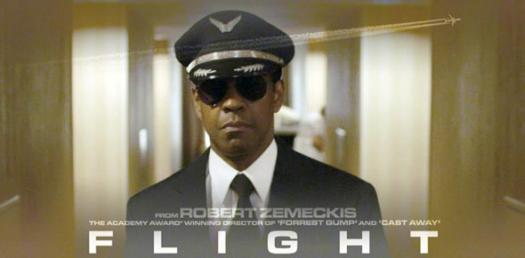# Variables And Flight

10 Questions | Total Attempts: 76SettingsMathematicians and lovers of all things that involve calculating the diameter of a circle or radius take this quiz. Get ready for your knowledge to be tested on different uses of variables and flight.

Related Topics
• 1.
David wound his FOSS plane rubber band 60 times, and the plane flew a length of 190 cm across a flat fight line. Then he used half as many twists of his rubber band and measured the distance it flew. What is a reasonable guess as to how far the plane might have flown the second time?
• A.

• B.

• C.

• 2.
What is the manipulated variable in David's experiment?
• A.

Distance flown

• B.

Number of rubber band twists

• C.

Angle (slope) of flight line

• 3.
What is the controlled variable in David's experiment?
• A.

Distance flown

• B.

Number of rubber band twists

• C.

Angle (slope) of flight line

• 4.
What is the responding variable in David's experiment?
• A.

Distance flown

• B.

Number of rubber band twists

• C.

Angle (slope) of flight line

• 5.
Which of these is NOT a variable in David's experiment?
• A.

Distance flown

• B.

Number of rubber band twists

• C.

Number of passengers (paper clips)

• 6.
Which of these is NOT a force associated with flight?
• A.

Thrust

• B.

Size

• C.

Drag

• 7.
Which of these forces opposes gravity (weight)?
• A.

Thrust

• B.

Drag

• C.

Lift

• 8.
Which of these forces opposes thrust?
• A.

Gravity

• B.

Drag

• C.

Lift

• 9.
A parachute causes which force?
• A.

Lift

• B.

Thrust

• C.

Drag

• 10.
In a controlled experiment to investigate what effect mass has on the distance a FOSS plane can fly, all of these variables should be controlled EXCEPT:
• A.

Number of rubber band winds

• B.

Number of passengers (paper clips)

• C.

Angle (slope) of the flight line